# Kriging : draw covariance models¶

import openturns as ot
import openturns.viewer as otv
from matplotlib import pylab as plt
import pylab as pl


## Abstract¶

Gaussian processes are a common fixture in UQ. They are defined by their covariance function and the library implements several of them. In this example we should depict covariance functions and play with parameters for two families of models: the generalized exponential model and the Matern models.

For visualization sake we should limit ourselves to the dimension 1.

dimension = 1


We set the lower bound to zero for stationary kernels

ot.ResourceMap.SetAsScalar("CovarianceModel-DefaultTMin", 0.0)


## The generalized exponential model¶

The GeneralizedExponential class implements a generalized exponential with a parameter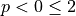exponent. The case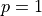is the standard exponential model while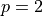is the squared exponential.

### Various parameters p and a fixed correlation length of 0.1¶

In this part we set the correlation length to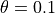and study three different models with parameters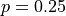,andand trajectories from gaussian processes based on these models.

We define thegeneralized exponential model :

covarianceModel = ot.GeneralizedExponential([0.1], 0.25)


We define thegeneralized exponential model :

covarianceModel2 = ot.GeneralizedExponential([0.1], 1.0)


We define thegeneralized exponential model :

covarianceModel3 = ot.GeneralizedExponential([0.1], 2.0)


We draw the covariance models :

graphModel = covarianceModel.draw()
graphModel.setColors(["green", "orange", "blue"])
graphModel.setXTitle(r"$\tau = \|s-t\|$")
graphModel.setYTitle(r"$C(\tau)$")
graphModel.setLegends([r"$p = 0.25$", r"$p = 1$", r"$p = 2$"])


For each covariance model we build a gaussian process and generate a random trajectory of on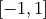. We first build a discretization of this interval with a regular grid with step 0.01.

xmin = -1.0
step = 0.01
n = 200
grid1D = ot.RegularGrid(xmin, step, n + 1)
nbTrajectories = 1


We define the first gaussian process and its trajectory :

process = ot.GaussianProcess(covarianceModel, grid1D)
sample = process.getSample(nbTrajectories)


then the second one and its trajectory :

process2 = ot.GaussianProcess(covarianceModel2, grid1D)
sample2 = process2.getSample(nbTrajectories)


and finally the third one and its trajectory :

process3 = ot.GaussianProcess(covarianceModel3, grid1D)
sample3 = process3.getSample(nbTrajectories)


We draw the trajectories :

graphTraj = sample.drawMarginal(0)
graphTraj.setXTitle(r"$x$")
graphTraj.setYTitle(r"$GP_{\nu}(x)$")
graphTraj.setTitle("Random realization from the covariance model")
graphTraj.setColors(["green", "orange", "blue"])
graphTraj.setLegends([r"$p = 0.25$", r"$p = 1$", r"$p = 2$"])


We present each covariance model and the corresponding tracjectory side by side.

fig = pl.figure(figsize=(12, 4))
_ = otv.View(graphModel, figure=fig, axes=[ax_pdf])
_ = otv.View(graphTraj, figure=fig, axes=[ax_cdf])
_ = fig.suptitle(r"Generalized Exponential Model : influence of the p parameter")The blue trajectory corresponding to the parameteris smooth as expected as compared with theprocess which is less regular.

### The exponential model ()¶

In the case of the exponential model () we show the influence of the correlation length on the trajectories.

with correlation length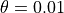:

covarianceModel = ot.GeneralizedExponential([0.01], 1.0)


with correlation length:

covarianceModel2 = ot.GeneralizedExponential([0.1], 1.0)


with correlation length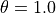covarianceModel3 = ot.GeneralizedExponential([1.0], 1.0)


We draw the covariance models :

graphModel = covarianceModel.draw()
graphModel.setColors(["green", "orange", "blue"])
graphModel.setXTitle(r"$\tau = \|s-t\|$")
graphModel.setYTitle(r"$C(\tau)$")
graphModel.setLegends([r"$\theta = 0.01$", r"$\theta = 0.1$", r"$\theta = 1$"])


For each covariance model we build a gaussian process and generate a random trajectory of on. We first build a discretization of this interval with a regular grid with step 0.01.

xmin = -1.0
step = 0.01
n = 200
grid1D = ot.RegularGrid(xmin, step, n + 1)
nbTrajectories = 1


We define the first gaussian process and its trajectory :

process = ot.GaussianProcess(covarianceModel, grid1D)
sample = process.getSample(nbTrajectories)


then the second one and its trajectory :

process2 = ot.GaussianProcess(covarianceModel2, grid1D)
sample2 = process2.getSample(nbTrajectories)


and finally the third one and its trajectory :

process3 = ot.GaussianProcess(covarianceModel3, grid1D)
sample3 = process3.getSample(nbTrajectories)


We draw the trajectories :

graphTraj = sample.drawMarginal(0)
graphTraj.setXTitle(r"$x$")
graphTraj.setYTitle(r"$GP_{\theta}(x)$")
graphTraj.setTitle("Random realization from the covariance model")
graphTraj.setColors(["green", "orange", "blue"])
graphTraj.setLegends([r"$\theta = 0.01$", r"$\theta = 0.1$", r"$\theta = 1$"])


We present each covariance model and the corresponding tracjectory side by side.

fig = pl.figure(figsize=(12, 4))
_ = otv.View(graphModel, figure=fig, axes=[ax_pdf])
_ = otv.View(graphTraj, figure=fig, axes=[ax_cdf])
_ = fig.suptitle(r"Exponential Model : influence of correlation length $\theta$")We observe a smoother trajectory with a high correlation value.

### The squared exponential ()¶

In the case of the squared exponential model () we show the influence of the correlation length on the trajectories.

with correlation length:

covarianceModel = ot.GeneralizedExponential([0.01], 2.0)


with correlation length:

covarianceModel2 = ot.GeneralizedExponential([0.1], 2.0)


with correlation lengthcovarianceModel3 = ot.GeneralizedExponential([1.0], 2.0)


We draw the covariance models :

graphModel = covarianceModel.draw()
graphModel.setColors(["green", "orange", "blue"])
graphModel.setXTitle(r"$\tau = \|s-t\|$")
graphModel.setYTitle(r"$C(\tau)$")
graphModel.setLegends([r"$\theta = 0.01$", r"$\theta = 0.1$", r"$\theta = 1$"])


For each covariance model we build a gaussian process and generate a random trajectory of on. We first build a discretization of this interval with a regular grid with step 0.01.

xmin = -1.0
step = 0.01
n = 200
grid1D = ot.RegularGrid(xmin, step, n + 1)
nbTrajectories = 1


We define the first gaussian process and its trajectory :

process = ot.GaussianProcess(covarianceModel, grid1D)
sample = process.getSample(nbTrajectories)


then the second one and its trajectory :

process2 = ot.GaussianProcess(covarianceModel2, grid1D)
sample2 = process2.getSample(nbTrajectories)


and finally the third one and its trajectory :

process3 = ot.GaussianProcess(covarianceModel3, grid1D)
sample3 = process3.getSample(nbTrajectories)


We draw the trajectories :

graphTraj = sample.drawMarginal(0)
graphTraj.setXTitle(r"$x$")
graphTraj.setYTitle(r"$GP_{\theta}(x)$")
graphTraj.setTitle("Random realization from the covariance model")
graphTraj.setColors(["green", "orange", "blue"])
graphTraj.setLegends([r"$\theta = 0.01$", r"$\theta = 0.1$", r"$\theta = 1$"])


We present each covariance model and the corresponding tracjectory side by side.

fig = pl.figure(figsize=(12, 4))
_ = otv.View(graphModel, figure=fig, axes=[ax_pdf])
_ = otv.View(graphTraj, figure=fig, axes=[ax_cdf])
_ = fig.suptitle(
r"Squared exponential model : influence of correlation length $\theta$"
)Execpt for very small values of the correlation length, trajectories are usually smooth. It is the main effect of the squared exponential model which leads to smooth process.

## The Matern covariance model¶

The MaternModel class implements the Matern model of parameter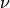. This parameter controls the smoothness of the process : for any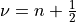the process is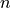times continuously differentiable.

### Influence of the regularity¶

In this paragraph we represent three models with different regularity and generate the corresponding random trajectories. We shall use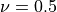,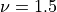and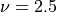and observe the regularity.

We define theMatern model :

covarianceModel = ot.MaternModel([1.0], 0.5)


We define theMatern model :

covarianceModel2 = ot.MaternModel([1.0], 1.5)


We define theMatern model :

covarianceModel3 = ot.MaternModel([1.0], 2.5)


We draw the covariance models :

graphModel = covarianceModel.draw()
graphModel.setColors(["green", "orange", "blue"])
graphModel.setXTitle(r"$\tau = \|s-t\|$")
graphModel.setYTitle(r"$C(\tau)$")
graphModel.setLegends([r"$\nu = 1/2$", r"$\nu = 3/2$", r"$\nu = 5/2$"])


For each covariance model we build a gaussian process and generate a random trajectory of on. We first build a discretization of this interval with a regular grid with step 0.001.

xmin = -5.0
step = 0.01
n = 1000
grid1D = ot.RegularGrid(xmin, step, n + 1)
nbTrajectories = 1


We define the first gaussian process and its trajectory :

process = ot.GaussianProcess(covarianceModel, grid1D)
sample = process.getSample(nbTrajectories)


then the second one and its trajectory :

process2 = ot.GaussianProcess(covarianceModel2, grid1D)
sample2 = process2.getSample(nbTrajectories)


and finally the third one and its trajectory :

process3 = ot.GaussianProcess(covarianceModel3, grid1D)
sample3 = process3.getSample(nbTrajectories)


We draw the trajectories :

graphTraj = sample.drawMarginal(0)
graphTraj.setXTitle(r"$x$")
graphTraj.setYTitle(r"$GP_{\nu}(x)$")
graphTraj.setTitle("Random realization from the covariance model")
graphTraj.setColors(["green", "orange", "blue"])
graphTraj.setLegends([r"$\nu = 1/2$", r"$\nu = 3/2$", r"$\nu = 5/2$"])


We present each covariance model and the corresponding tracjectory side by side.

fig = pl.figure(figsize=(12, 4))
_ = otv.View(graphModel, figure=fig, axes=[ax_pdf])
_ = otv.View(graphTraj, figure=fig, axes=[ax_cdf])
_ = fig.suptitle(r"Matern model : influence of the regularity $\nu$ parameter")The red trajectory is the least regular (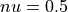) as it is only continuous. We see that the the blue trajectory is more smooth as expected.

### Variation of the correlation length¶

In this paragraph we fix the regularity by choosingso we expect a continuously differentiable realization. We then use three different correlation lengths,andand observe the impact on realizations of gaussian processes based on these covariance models.

We define the Matern model with:

covarianceModel = ot.MaternModel([0.01], 1.5)


We define the Matern model with:

covarianceModel2 = ot.MaternModel([0.1], 1.5)


We define the Matern model with:

covarianceModel3 = ot.MaternModel([1.0], 1.5)


We draw the covariance models :

graphModel = covarianceModel.draw()
graphModel.setColors(["green", "orange", "blue"])
graphModel.setXTitle(r"$\tau = \|s-t\|$")
graphModel.setYTitle(r"$C(\tau)$")
graphModel.setTitle("Matern covariance model with \nu = 3/2")
graphModel.setLegends([r"$\theta = 0.01$", r"$\theta = 0.1$", r"$\theta = 1.0$"])


For each covariance model we build a gaussian process and generate a random trajectory of on. We build a discretization of this interval with a regular grid with step 0.01.

xmin = -1.0
step = 0.01
n = 200
grid1D = ot.RegularGrid(xmin, step, n + 1)
nbTrajectories = 1


We define the first gaussian process and its trajectory :

process = ot.GaussianProcess(covarianceModel, grid1D)
sample = process.getSample(nbTrajectories)


then the second process :

process2 = ot.GaussianProcess(covarianceModel2, grid1D)
sample2 = process2.getSample(nbTrajectories)


and the third one :

process3 = ot.GaussianProcess(covarianceModel3, grid1D)
sample3 = process3.getSample(nbTrajectories)


We draw the trajectories :

graphTraj = sample.drawMarginal(0)
graphTraj.setXTitle(r"$x$")
graphTraj.setYTitle(r"$GP_{\theta}(x)$")
graphTraj.setColors(["green", "orange", "blue"])
graphTraj.setLegends([r"$\theta = 0.01$", r"$\theta = 0.1$", r"$\theta = 1.0$"])


We present each covariance model and the corresponding tracjectory side by side.

fig = pl.figure(figsize=(12, 4))
_ = otv.View(graphModel, figure=fig, axes=[ax_pdf])
_ = otv.View(graphTraj, figure=fig, axes=[ax_cdf])
_ = fig.suptitle("The Matern model : variation of the correlation length")From the previous figure we see that the trajectory of the gaussian process is smoother with large correlation length.

Display figures

plt.show()


Reset default settings

ot.ResourceMap.Reload()